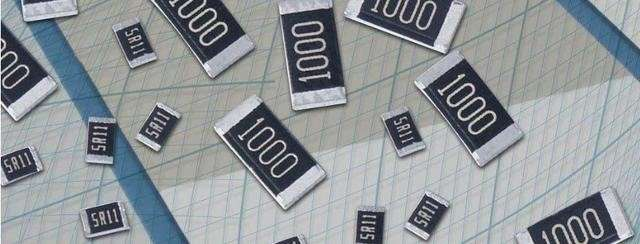# How to Read Chip Resistor ?【Synton-Tech】

## How to Read Chip Resistor ?

With the continuous development of new technologies, more and more products need to use resistors, making the types of resistors more and more. The main ones are: chip resistors, thin film and thick film resistors, metal film resistors, carbon film resistors, Winding resistance, etc. Chip resistors in the circuit have the advantages of small size, light weight, stable electrical performance, high reliability, low assembly cost, high mechanical strength, high-frequency characteristics, etc.; due to these characteristics, we can see chip resistors in many circuits The use of, usually has a string of numbers on its surface, which represents some resistance performance of the chip resistor.

So how to read the numbers of chip resistance? The more resistance, the more difficult it is to distinguish its resistance, accuracy, power, material, etc. The following editor will introduce you all:

Resistance conversion relationship Ω=Ωk=kΩ=1,000ΩM=MΩ=1,000,000Ω Thecode on the micro-chip resistor is generally marked with 3 digits or 4 digits...

Resistance conversion relationship

Ω = Ω
k = kΩ = 1,000 Ω
M = MΩ = 1,000,000 ΩResistance conversion
The identification of the resistance value of the chip resistor is actually very simple. The resistance value of the chip resistor is printed on the surface. When reading the resistance value of the chip resistor, you can directly look at the number on the surface of the resistor; the chip resistance is composed of three numbers, which means the resistance error is ±5 %. The first two digits are significant digits, and the third digit indicates the multiplying rate by zero. The basic unit is Ω. For example, 102, 1 and 0 are significant digits and just write them down directly, 2 means multiplying by zero, that is, the power of 10 (simply put, the third digit is what is the power of 10)
To
We can easily understand the chip resistance identification method through the following examples:
10×1000=10000Ω=10KΩ
103=10×1000=10000Ω=10KΩ
223=22×1000=22000Ω=22KΩ
122=12×1000=1200Ω=1.2KΩ
301=30×10=300Ω
850=85×(zero power of 10=1)=85Ω
1R0=1.0Ω

2. The chip resistor is composed of four numbers, indicating that the error of the resistance is ±1%. The first three digits are significant digits, and the fourth digit represents the multiplication by zero (that is, the number is how many times it represents the power of 10). For example, 1502, 150 are valid digits, just write them down, 2 means 10 squares.
So the resistance of 1502 is 150×10 squared = 150×100=15000Ω=15KΩ

3. To read the resistance value of the chip resistor composed of numbers and letters, we only need to change R to a decimal point.
10R6=10.6R=10.6Ω R12=0.12R=0.12Ω

 1R1=0.1Ω R22=0.22Ω R33=0,33Ω R47=0.47Ω R68=0.68Ω R82=0.82Ω 1R0=1Ω 1R2=1.2Ω 2R2=2.2Ω 3R3=3.3Ω 2R7=4.7Ω 5R6=5.6Ω 6R8=6.8ΩΩ 8R2=8.2Ω 100=10Ω 120=12Ω 150=15Ω 180=18Ω 220=22Ω 270=27Ω 330=33Ω 390=39Ω 470=47Ω 560=56Ω 680=68Ω 820=82Ω 101=100Ω 121=120Ω 151=150Ω 181=180Ω 221=220Ω 271=270Ω 331=330Ω 391=390Ω 471=470Ω 561=560Ω 681=680Ω 821=820Ω 102=1KΩ 122=1.2KΩ 152=1.5KΩ 182=1.8KΩ 222=2.2KΩ 272=2.7KΩ 332=3.3KΩ 392=3.9KΩ 472=4.7KΩ 562=5.6KΩ 682=6.8KΩ 822=8.2KΩ 103=10KΩ 123=12KΩ 153=15KΩ 183=18KΩ 223=22KΩ 273=27KΩ 333=33KΩ 393=39KΩ 473=47KΩ 563=56KΩ 683=68KΩ 823=82KΩ 104=100KΩ 124=120KΩ 154=150KΩ 184=180KΩ 224=220KΩ 274=270KΩ 334=330KΩ 394=390KΩ 474=470KΩ 564=560KΩ 684=680KΩ 824=820KΩ 105=1MΩ 125=1.2MΩ 155=1.5MΩ 185=1.8MΩ 225=2.2MΩ 275=2.7MΩ 335=3.3MΩ 395-3.9MΩ 475=4.7MΩ 565=5.6MΩ 685=6.8MΩ 825=8.2MΩ 106=10MΩ